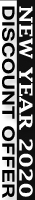0% Plagiarism Guaranteed & Custom Written

# QNM444 - Quantitative Approaches to Decision Making

Subject Title
Quantitative Approaches to Decision Making

Subject Description
This subject applies the basic knowledge of statistics developed in QNM222 to several important decision-making applications including hypothesis testing, regression and correlation analysis, linear programming, and time series.

Learning Outcomes
Upon successful completion of this subject the student will be able to:

1. Formulate hypothesis tests, estimate or make decisions about population means or proportions based on one and two sample(s) selected from a population.

2. Apply simple and multiple linear regression analysis, i.e., relate the mean value of a single dependent variable to one or more independent variables.

3. Describe the behaviour of a variable by analyzing a time series of that variable.

4. Calculate the expected value of perfect and sample information.

5. Calculate the optimal solution to a business decision given an objective and a set of resource constraints.

6. Prepare written interpretations of the results of various statistical analysis.

7. Apply spreadsheet software (EXCEL) to solve statistical problems.

100% Plagiarism Free & Custom Written,